MATH 106  Calculus for Business and Economics    Spring, '00
Class Summaries/Outlines

 2/7 2/8 2/9 2/10 3/6 3/7 3/8 3/9 4/3 4/3 4/4 4/5 5/1 5/2 5/3 5/4 1/19 1/20 2/14 2/15 2/16 2/17 3/13 3/14 3/15 3/16 4/10 4/11 4/12 4/13 1/24 1/25 1/26 1/27 2/21 2/22 2/23 2/24 3/20 3/21 3/22 3/23 4/17 4/18 4/19 4/20 1/31 2/1 2/2 2/3 2/28 2/29 3/1 3/2 3/27 3/28 3/29 3/30 4/24 4/25 4/26 4/27
• 1/19 Numbers, Variables, Algebra Review 1.1&1.2
• Go over Background Analysis.
• Solutions:
1. B: y=2x+5
2. D: -9/10
3. A:  (x-1)/x^3
4. D:  x^2/(3y^9)
5. D:  3x + 2 sqrt(15xy) + 5y
6. C:  \$52
7. D: 4
• SECTION 1.1
• Real numbers are decimals (possibly infinite and non-repeating)
• The number line. Points on the line correspond to real numbers.
• Absolute values.
• Example:  |-2|=2;  |sqrt(5)-5| = 5-sqrt(5).
• Example*:  |2 + - 3| < |2| + |-3|
• Equality, inequality, and intervals.
• Solving a linear equation.
• Example.  3x+5=29:

• 3x= 24;  x =8.... check.
• Solving a linear inequality.
• Example:   3x+5<29:

•   3x<24;    x<8...  x in the interval (-inf,8).
(No total check- but some confirming evidence!)
• Example:   -2x < 10:

•     x > -5 .... x in the interval (-5, inf).
• integer exponents:
• 2^3 = 2*2*2 = 8
• 2^0 = 1
• 2^-3 =1/ (2^3) = 1/8
• fraction exponents and roots
• 8^(1/3) = cube root of 8 = 2
• Properties:
• SAME BASE: Products, quotients, powers
• SAME POWER: Products. quotients.

•
• SECTION 1.2
• Multiplication and Factoring:
• a*(b+c)= a*b + a*c
• quadratic expressions (see text p 17)

•
• 1/20
• Class spent much time on problems from the review sheet related to functions.

• Function notation was reviewed. Mapping(transformation) figures and graphs of functions were introduced .
2. For this problem let f be defined by f(x) = 5x2 + 3.
A. Find the following. Simplify you answer when possible.
i) f(1)  = 5(1)2 + 3=8                    iii) f(1+h)= 5(1+h)2 + 3= 8+10h+5h2
ii) f(h)  =  5h2 + 3        iv) [f(1+h)-f(1)]/h = [10h +5h2 ]/h=h[10+5h]/h= 10 +5h
B. Find any number(s) z where f(z) = 23.
f(z)= 5z2 + 3= 23 ;     5z2 =20;   ... z = 2  OR z = -2.
C. For which values of x is f(x) < 23? Express your answer as an interval.
f(x)= 5x2 + 3 < 23; so  5x2 < 20;   x2 < 4; so -2<x<2... or x is in the interval (-2,2).

5. Boyle's law states that , for a certain gas P*V = 320, where P is pressure and V is volume.
A. Draw a complete graph representing this situation.  Label your axes and write an equation for each asymptote.
Make a table of values for P and V using P=320/V and P>0, V>0.

 V P 80 4 160 2 320 1 640 .5

Now use these data points to sketch the graph.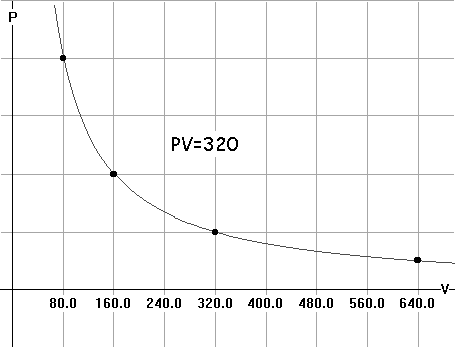B. If 8 < V < 40, what are the corresponding values of P?
Notice that when V=8, P = 40 and when V=40, P= 8. So as V varies from 8 to 40, P varies from 40 to 8.

• We also considered problem 115 on page 13.

• C: the monthly commission. X: the total sales .
C= .15 (X-12000) >= 1000; so X-12000>=1000/.15  and thus X >= 12000 + 1000/.15 = 12000 +20000/3= \$18667
• 1/24 start here:
• Summary of Chapter 1 in Calculus Applied to the Real World (on-line)
• We did several problems from 1.1 and 1.2... including problems about fractional and negative exponents, and factoring to solve a quadratic equation.
• [Also Reviewed interval notations]
• Roots and equations.
• Example:  x^2-5x + 6 = 0 :

•   Factor (x-3)*(x-2)= 0
so either x-3=0 or x-2 = 0.
Thus x=3 or x=2 are solutions. CHECK!
• Example:  x^2-5x + 6 = 0 :

• x=[-(-5)+ or- sqrt( (-5)^2-4*1*6)]/2*1
=[ 5 + or - sqrt(25-24)]/2
= [5 + or - 1]/2
= 6/2 or 4/2
so x = 3 or 2.
• Fractions  (Rational Expressions)
• Factor and "cancel"
• Common Denominators [Still to be reviewed.]
• Rationalizing expressions with roots. [Still to be reviewed.]

•
• SECTION 1.3
• Cartesian Coordiantes
• Distance
• Example: eq'n of a circle with center at (1,2) and radius 3.

•
• SECTION  1.4
• Slope
• m =  (y2- y1)/ (x2-x1)
• Example: find slope of line through the points (4, 2) and (1,3):

• slope= m = (3-2)/(1-4) = 1/(-3) = - 1/3
• Interpret: m>0; m<0; m=0
• parallel non-vertical lines have the same slope.

•
• 1/25
• Start with a review of lines, then go on to functions (2.1)
• Equations for lines:
• Vertical:
• Example:X=3
• Non-vertical:
•  Point- slope
• Example: m=5 through (2,3):

• Y-3=5(X-2)   or   Y=5(X-2)+3
•  slope- Y intercept
• Example: m=5 Y intercept is (0,7)

• Y=5X + 7
• General Equation:
• Example: find X and Y intercepts for 2X + 3Y - 6 = 0:

• Set X= 0;   3Y=6;   Y=2   so (0,2) is the Y intercept.
Set Y=0;    2X=6;   X=3  so (3,0) is the X intercept.
• SECTION 2.1   FUNCTIONS.
• What is a function?
• A function f is a correspondence (rule) which assigns to each member of the domain (source) set, x, a member of the co-domain (target) set, denoted f(x) .
• How are functions defined?
• with a formula.

• The function g is defined by assigning to the number x the value x2+5. This is expressed by the equation: g(x)=x2+5.

With this definition we have
g(0)=5, g(1)=6, g(-1)=6,g(2)=9, g(1/2)=21/4, g(t)=t2+5, g(1+t)=(1+t)2+5, etc.

• with a table.

•
 x f(x) 2 5 1 3 0 -2 -1 4 -2 0
• with a graph.

•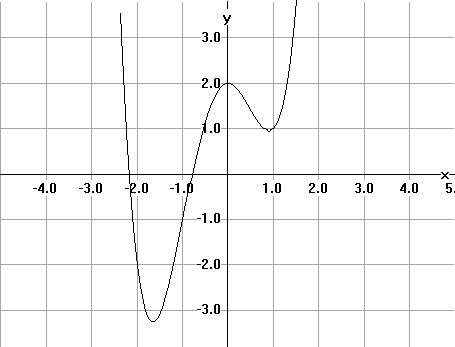The vertical line test: A graph measuring variables X and Y determines Y as a function of X if  each vertical line meets the graph at at most one point.
• with a context.

• Suppose that C is the cost of producing X liters of OURBEST wine. C is a function of X
• How are functions visualized?
• Mapping figures/transformation figures.

•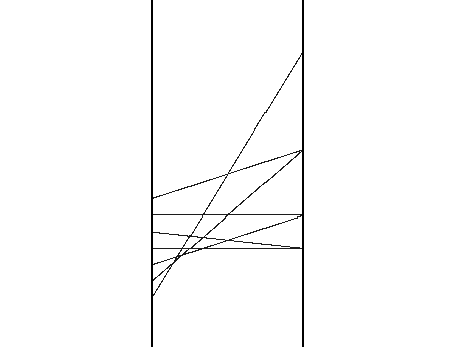• Graphs.
• 1/26
• Problem #8 was done !

•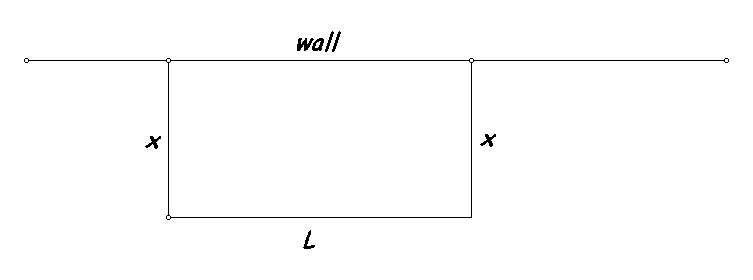The wall is 200 meters long. We have 140 meters of fence. This is a constraint. In terms of the lengths measured x and L we have that 2x+L = 140.  Thus L = 140 -2x.
The area, A, is found by multiplying x by L.  Thus A = x (140-2x) = 2x(70-x). We made a table of some values of this function.  We noted that 0<=x<=70.

 x L A 10 120 1200 20 100 2000 70 0 0 60 20 1200 50 40 2000
• We looked at the transformation figure from this data as well as the graph.

• This led to a conjecture from the symmetry that the largest value for the area, A, would occur when x = 35. in this case A=35*70=2450.

In fact, A = -2x2+140x  but A also can be expressed by A= -2(x-35)2 +2450. It should be clear from thinking about this expression for A that A is at most 2450, since for any x other than 35, A will be the result of subtracting a positive number from 2450.

• We then examined this function's graph using WINPLOT.

•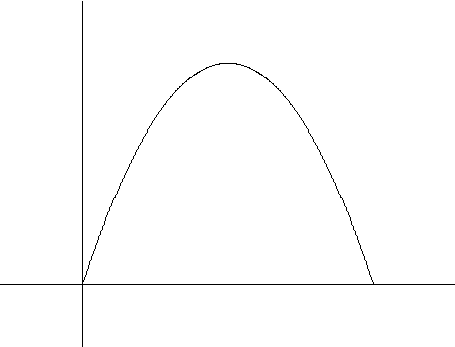• At the end of class we looked briefly at two problems on functions and transformation figures.
• 1/27
• We looked at problems from today's and the previous day's assignment.

• Using these problems we reviewed several aspects of functions as indicated below by &&.
• More on functions and Mathematical Modeling. (2.1 and 2.3)

• Use Winplot to graph examples of these with parameters.
• More examples of functions.

• f(x)= Ax2 + Bx + C
• && Functions defined in cases.

• i. s(x)= 2x+1 when x <3 ; -x+10 when x>=3
ii s(x)= 2x+1 when x <3 ; Ax+B when x>=3

• Polynomial functions.

• cubic c(x)= Ax3 +Bx 2 + Cx + D
quartics q(x)= Ax4 + Bx + Cx 2 +Dx +E

• && Rational functions.

• R(x)=f(x)/g(x) where f and g are polynomials.
Examples: use polynomials above for f and g.

Example: Average cost= C(x)/x

• && Power/root functions.

• examples: f(x)=sqrt(x); g(x)= x1/3
• 1/31
• Finish a few details on functions- especially  considering equilibrium for supply/demand.
• Demand   p =f( xd)  N.B. This reverses the more sensible relationship as xd being a function of p.
• Supply   p =f( xs).  N.B. This also reverses the more sensible relationship as xs being a function of p.

•
Use Winplot to graph examples.
• Begin Rates and slopes and margins.

•
• average velocity (rate), slope of a secant line, marginal cost/unit for an indivisible commodity.

•

Average Velocity Example: Suppose position of an anvil falling from a 100 foot cliff is a function of time,
S = -16t2 +100 feet at time t seconds.
The position at times t=1 and t=2.
Find the average velocity during the time interval [1,2].

Solution: S(1) = -16+100 = 84;  S(2) = -16*4 + 100 =  36.
Average velocity = change in position/ change in time = [S(2)-S(1)]/[2-1] =  (36-84)/(2-1) = -48 ft/sec.
Note: The negative velocity indicates the anvil is falling down. The average speed of the anvil is 48  ft/sec.

• 2/1

•
Use Winplot to graph examples.
• Instantaneous velocity, slope of a tangent line; marginal cost/unit for a divisible commodity .
• Average velocity-Instantaneous velocity
• Average rate of price change wrt time- instantaneous rate of price change wrt time
• average marginal cost per unit- marginal cost per unit
• slope of secant line to curve- slope of tangent line to a curve.
• Slopes of tangents and secants, instantaneous rates and average rates. Estimation and limits.
• Suppose Y= f(X).  Consider X=a and X=a+h.  These correspond to 2 points on the the graph of f,

• (a, f(a)) and (a+h, f(a+h)).  The line connecting these two points ids called the secant line.

The slope of the secant line is [f(a+h)-f(a)]/h.

This is also the average velocity of an object moving on a straight line with its position S = f(t) at time t for the time interval between time time t= a and time t=a+h.

• When h is very close to 0, h  -> 0,

• the value of [f(a+h)-f(a)]/h will approximate the slope of the line tangent to the graph of f at the point (a,f(a).  It will also approximate the instantaneous velocity of the object moving on the straight line at time t=a.

• Example: f(x)= 100-16x2.  Find the slope of the tangent to the graph at when x=2.

• Solution: . First find the slope of the secant line  determined by X=2 and X= 2+h.
f(a)=100-16a; a =2 so f(2) = 100 - 16*4= 36.
f(a+h)= f(2+h) = 100 -16(2+h) 2 = 100 - 64 - 64h -16h2 = 36 -64h -16 h2 ;
Thus f(a+h) - f(a) = -64h -16h2  = h(-64-16h) and [f(a+h)-f(a)]/h = -64 -16h.

Now: THINK! When h ->0,  this slope = -64-16h -> -64. Thus the slope is -64.

• Example: Suppose S =f(t) feet where t is measured in seconds with f from the previous example. Then the instantaneous velocity of the object at time 2 seconds is -64 feet per second.
• 2/2
•
• Continued discussion of the tangent line/instantaneous velocity/rate problem.
• The equation of the tangent line when x=2 is given by

• y=-64(x-2) + 36.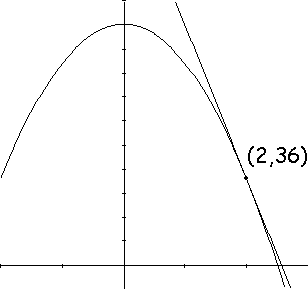• To find the slope of the tangent at a more general contect when x=a:

• Consider the quotient [f(a+h)-f(a)]/h  using the general choice of a in place of the specific value x=2:
f(a+h) =100 - 16(a+h)2  = 100 -16a2  -32ah-16h2
f(a)   =100 - 16 a2
so
f(a+h)-f(a)= -32ah - 16h2  = (-32a-16h)h
and thus [f(a+h)-f(a)]/h= -32a -16h.
Now considering what happens when h is close to 0, we have the slopes of the secants =-32a-16h  -> -32a.
• This is consistent with the result when x=2.

•
• Limit notation for expressing this work:....
• Do this for x...

•
• 2/3 See the text chapter 2 summary and Section 3.1 for examples of what we did today.
• We talked more about the definition of the derivative and finding the derivative using algebra and thinking.
• We started an examination of the calculus of derivatives using constants. powers, constant multiples,  and sums.
• We started to use Leibniz notation for the derivative.

•
• 2/7 More on the rules of the calculus for derivatives.
• Justifications for some rules: constants, powers (integers, square roots), constant multiples, sums.
• Start product and quotient rules.
• 2/8 More on the rules of the calculus for derivatives.
• Justifications and interpetations of the derivatives of powers, constant multiples and sums.
• Introduced Product Rule.

•
• 2/9 More on the rules of the calculus for derivatives.
• Demonstration of the product rule's proof.
• Quotient rule introduced and justified based on the product rule.
• 2/10 More on the rules of the calculus for derivatives.
• More work with the quotient rule.
• If  Q(x) = 1/ P(x) then by applying the quotient rule we have

• Q'(x)= -P'(x)/[P(x)]2.
• If  f(x) = 1/ x n  = x -n then f '(x) = -nx n-1 / x 2n = -n x -n-1.
• If C(x) = the total cost for producing x units, C'(x) is the marginal cost for producing one more unit.

• If we denote by C (x) = C(x)/x = the average cost for producing x units, then by the quotient rule C '(x) = [x C'(x) -C(x)]/x 2  is the marginal average cost for producing one more unit.
• Using time, t , position of a car, s , and the consumption of gas, g, during a trip we  started a consideration of the chain rule: dg/dt = dg/ds * ds/dt.

•
• 2/14 Class cancelled (power failure)
• 2/15 More on the chain rule. Marginal cost, reveues, and profit.
• 2/16
• Higher order derivatives.
• Implicit differention.
• 2/17
• Related Rates
• The differential
• Elasticity of demand.
• Suppose x= f(p). We wish to consider how a small change in price, h,  effects the change in quantity demanded on a percentage basis... i.e.

•  [Percent change in quantity demand] /  [ Percent change in unit price]
or [ {f(p+h) - f(p) }/f(p) *100] /  [ h/p *100]  .
When h is small this is approximately f '(p) /(f(p)/p) =  p*f '(p) / f(p).
• The elasticity of demand is  defined to be E(p) =  -p*f '(p) / f(p) = - p / x * dx/dp.